# Fractional-linear function

A function of the type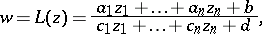where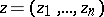are complex or real variables,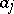,,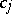,are complex or real coefficients, and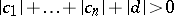. If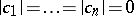, the fractional-linear function is an integral-linear function; if the rank of the matrix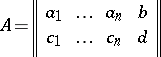is equal to one,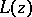is a constant. A proper fractional-linear function is obtained if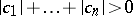and if the rank ofis two; it assumed in what follows that these conditions have been met.

Ifand,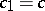,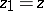are real, the graph of the fractional-linear function is an equilateral hyperbola with the asymptotes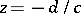and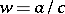. Ifand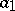,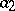,,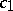,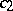,,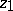,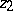are real, the graph of the fractional-linear function is hyperbolic paraboloid.

If, the fractional-linear functionis an analytic function of the complex variableeverywhere in the extended complex plane, except at the pointat whichhas a simple pole. If, the fractional-linear functionis a meromorphic function in the spaceof the complex variable, with the setas its polar set.